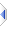# ceil

 Definition: atom x2 = ceil(atom x1) Description: Return the smallest integer greater than or equal to x1. (Round up to an integer, towards +infinity.) Comments: For non-integers, ceil() is one more than floor(), and for integers ceil() returns the same result as floor(), namely the input value unaltered. The result is rounded towards plus infinity. Note that while trunc() rounds towards zero, there is no complimentary builtin function that rounds negative fractions away from zero. This function may be applied to an atom or sq_ceil() to all elements of a sequence. The rules for sequence operations apply. Example: ```r = ceil(1.4) -- r is 2 r = ceil(-1.4) -- r is -1 ``` Implementation: See builtins\pmaths.e (an autoinclude) for details of the actual implementation. See Also: floor, trunc